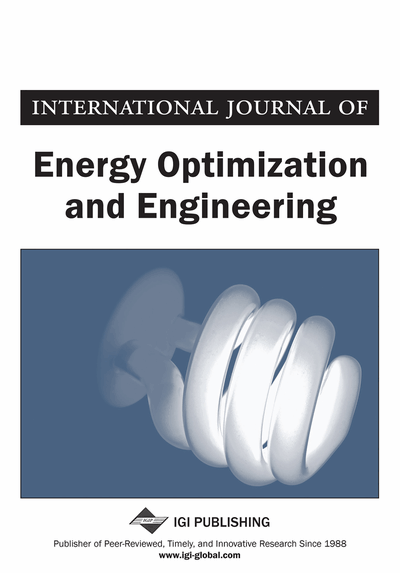# On the Exergy Content of an Isolated Body in Thermodynamic Disequilibrium

Robert W. Grubbström (Linköping Institute of Technology, Sweden and Mediterranean Institute for Advanced Studies, Šempeter pri Gorici, Slovenia)
DOI: 10.4018/ijeoe.2012010101
Available
\$37.50
No Current Special Offers

## Abstract

Exergy is a concept that is gaining an increasingly wider recognition as a proper measure for the actual energy resources consumed, when energy is used. Energy as such is indestructible, but exergy is not. As entropy is generated while energy is used, exergy is consumed. Exergy can be interpreted as the qualitative content of energy, or as energy in its highest quality. Therefore, there is an interest in investigating this concept from as many theoretical aspects as possible. In earlier papers the author has developed formulae for the exergy potential of a system of finitely extended objects, not necessarily having any environment. It was shown that the classical formula for exergy obtains as one of the objects grows beyond all bounds thereby taking on the rôle as an environment. In this current paper formulae are derived for the exergy content of an isolated body in thermodynamic disequilibrium, viewed as a system of infinitely many objects each with infinitesimal extension and in microscopic equilibrium.
Article Preview
Top

## 1. Introduction

The term exergy was coined in 1956 by Zoran Rant (1904-1972), (Rant, 1956). Earlier terms for this concept have been available energy and availability, inter alia (Kline, 1999). For a bibliography on exergy-related work, see Wall (1997). For arguments supporting the further development and application of this concept, please see e. g., Rosen et al. (2008).

Exergy may be defined as the maximum amount of mechanical work that can be extracted from a source with given properties. The original concept relies on the existence of an environment that has constant intensive properties (temperature, pressure, chemical potentials, etc.), which are not affected by the processes taking place during the extraction. If a maximal amount of mechanical work is to be extracted, the processes involved need to be ideal in the sense that no entropy is generated, i.e., the processes are reversible.

The main development in this paper is based on the following universal principle (Grubbström, 1985):

“The exergy of a system of objects equals its total inner energy less the inner energy of a single body having the same total extensive properties as those of the system.”

The new basic results in this article are obtained from reinterpreting an object in disequilibrium as a system of infinitely many infinitesimal objects with heterogeneous intensive properties. These results are summarised in the single formula (21) in Section 4. By “thermodynamic disequilibrium” we thus mean that the object under consideration on a macroscopic level has a heterogeneous distribution of at least one intensive property (such as temperature or pressure). An object in equilibrium has all its intensive properties at constant levels throughout.

We summarise classical developments in Section 2, followed by formulae for the exergy of a set of finite objects in Section 3. Our new considerations are mainly developed in Section 4, in which a finite object in disequilibrium is interpreted as a continuous set of infinitely small objects, each in microscopic equilibrium, but with different intensive properties in the finite perspective. Section 5 offers three simple examples illustrating our findings, and a final section contains a summary and some ideas for further developments. The concept of microscopic equilibrium is elaborated in Section 4.

In our treatment, we make use of two abstract spaces, on the one hand the thermodynamic configuration space erected by one axis for each thermodynamic property considered, on the other, the geometric space in which the object is physically embedded, the latter space erected by axes representing spatial co-ordinates.

Top

## 2. The Inner Energy Function And Classical Exergy Expression

Table 1.
Basic notation
 U Inner Energy Function [J] S Entropy [J/K] V Volume N Molar content [mole] T Absolute temperature [K] p Pressure [J/(volume unit)] μ Chemical potential [J/mole]Gradient of function f n Number of (extensive/intensive) properties of thermodynamic system, dimension of thermodynamic configuration space x Vector of extensive properties, n-dimensional y Vector of intensive properties, n-dimensional m Number of objects (sources),Vector of extensive properties of object i, i = 1, 2, …mVector of intensive properties of object i, i = 1, 2, …m E Exergy [J] a* Equilibrium value of property a, equilibrium value of intensive property in case of infinite environment c Specific heat capacity at constant volume [J/(mole·K)] R Universal gas constant [J/(mole·K)]Density (per unit of volume) of extensive property a,Vector of densities of extensive propertiesSpatial coordinatesVector of spatial coordinates

## Complete Article List

Search this Journal:
Reset
Volume 11: 4 Issues (2022): 1 Released, 3 Forthcoming
Volume 10: 4 Issues (2021)
Volume 9: 4 Issues (2020)
Volume 8: 4 Issues (2019)
Volume 7: 4 Issues (2018)
Volume 6: 4 Issues (2017)
Volume 5: 4 Issues (2016)
Volume 4: 4 Issues (2015)
Volume 3: 4 Issues (2014)
Volume 2: 4 Issues (2013)
Volume 1: 4 Issues (2012)
View Complete Journal Contents Listing